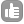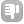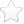2Hi, regarding my C++/Qt NMR processing application (see http://qa.nmrwiki.org/question/149/problem-reading-and-transforming-vendor-data-using-cqt or http://cutenmr.sourceforge.net/) I have a question concerning the window functions. I did research in the literature but could not find the "correct" mathematical formulas of the important window functions (exponential, gaussian, sine, sine squared, TRAF). For the gaussian function I found: exp(-(t*LB)^2) but also: exp(-LB*t^2). When coding my application I found out that neither can be correct because the result looks wrong. Even the simple exponential function seems to be a bit more complex than just: exp(-LB*t), because I noticed that I always had to enter a larger LB-value than intended in order to get a result that is comparable to other processing applications. I would be very thankful if someone could either tell me what the exact formulas are or point me to a reliable source where I can find them. Many thanks for any help :) asked Sep 19 '10 at 08:072For pure Gaussian, remenber that it is often defined by the width at 1 standard deviation rather than half-height. Also remember that the GM function in Brukerese is a Lorentz to Gauss transformation, not a pure Gaussian apodization function. Do you want me to send you the code that SpinWorks uses for these functions? answered Sep 21 '10 at 09:31Kirk Marat 711 Thanks for pointing these confusing differences in nomenclature/definition out. Sending me the corresponding code is a generous offer, this would help me a lot. Many many thanks for that. - Pascal Fricke (Sep 22 '10 at 01:14) O.K. Will try to send the code out to you later today or tomorrow. - Kirk Marat (Sep 22 '10 at 09:40)1An exponentially decaying `FID` where amplitude is described by `e^(-kt)` after `FT` will give a peak with width at half maximum of `k/pi`. So if you want to broaden the line by `LB` Hz you'll have to make `k=pi*LB` and the apodization formula will be `e^(-pi*LB*t)` answered Sep 21 '10 at 10:59Evgeny Fadeev 577●1 Many thanks for this answer. This explains the fact that the effect of the line broadening I observed was always too small. - Pascal Fricke (Sep 22 '10 at 01:07)0Try to find what is the unit in which LB parameter is defined: Is it in units of time or its reciprocal which is frequency. Using a parametr value in a equation without knowing what the units are to be might create the complication in the appearance of the results. Or other wise, which ever Parameter value is used for LB, and when this value in the equation does not seem right, then simply use the same parameter value in the denominator and compare the result. S.Aravamudhan answered Oct 18 '10 at 00:09